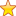# Square Root of Prime Number using Command Line Argument

The square root of a Prime number by checking first if it is a prime number?

Write a C program which will check whether a given number N is a Prime or Not. If the Number N is a Prime, then find it’s square root and print that value to the STDOUT as floating point number with exactly 2 decimal precision.
If the number is not Prime, then print the value 0.00 to STDOUT.
The given number will be positive non zero integer and it will be passed to the program as first command line argument.
Other than floating point No other information should be printed to STDOUT.

Also, you can study other Command Line Programming Questions here on our TCS Dashboard.

It is highly advisable to go through Command Line Arguments Post before even looking at the code. Please study this for TCS and come back to this post later.

[code language=”cpp”]
#include<stdio.h>
#include<stdlib.h>
#include<stdbool.h>
#include<math.h>
bool isPrime(int n)
{
if(n<2)
return false;
int i;
for(i=2;i*i<=n;i++)
{
if(n%i==0)
return false;
}
return true;
}
int main(int argc, char *argv[])
{
if(argc==1)
{
printf("No arguments");
return 0;
}
else
{
int n;
n=atoi(argv);
float sq=0;
if(isPrime(n))
{
sq=sqrt(n);
printf("%.2f",sq);
}
else
printf("%.2f",sq);
return 0;
}
}
[/code]

Summary
Review Date
Reviewed Item
TCS Square Root Program
Author Rating
5•02